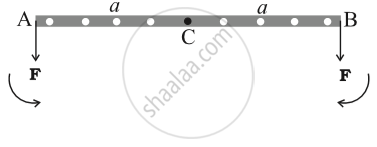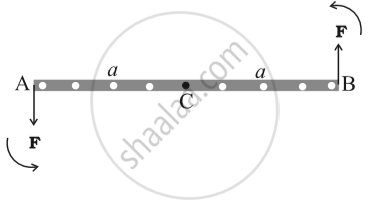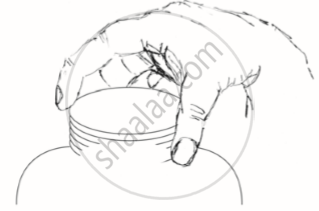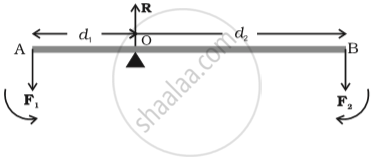# Equilibrium of Rigid Body

#### description

• Principle of moments
• Centre of gravity

### Equilibrium of Rigid Body

• A force changes the translational state of the motion of the rigid body, i.e. it changes its total linear momentum.

• A torque changes the rotational state of motion of the rigid body, i.e. it changes the total angular momentum of the body

Note: Unless stated otherwise, we shall deal with only external forces and torques.

A rigid body is said to be in mechanical equilibrium, if both its linear momentum and angular momentum are not changing with time. This means

Total torque should be zero => Rotational EquilibriumFnet = 2F
τnet = 0

• Total force should be zero => Translational EquilibriumFnet = 0
τnet = Fl

A pair of equal and opposite forces with different lines of action is known as a couple or torque. A couple produces rotation without translation.

When you open the lid of a jar, you apply couple on it.An ideal lever is essentially a light rod pivoted at a point along its length. This point is called the fulcrum

The lever is a system in mechanical equilibrium.Mechanical advantage = "F1"/"F2" = "d1"/"d2"

Mechanical advantage greater than one means that a small effort can be used to lift a large load.

Centre of Gravity

• The centre of gravity of a body is that point where the total gravitational torque on the body is zero.

• The centre of gravity of the body coincides with the centre of mass in uniform gravity or gravity-free space.

• If g varies from part to part of the body, then the centre of gravity and centre of mass will not coincide.

If you would like to contribute notes or other learning material, please submit them using the button below.

### Shaalaa.com

Rotational equilibrium [01:10:45]
S
0%